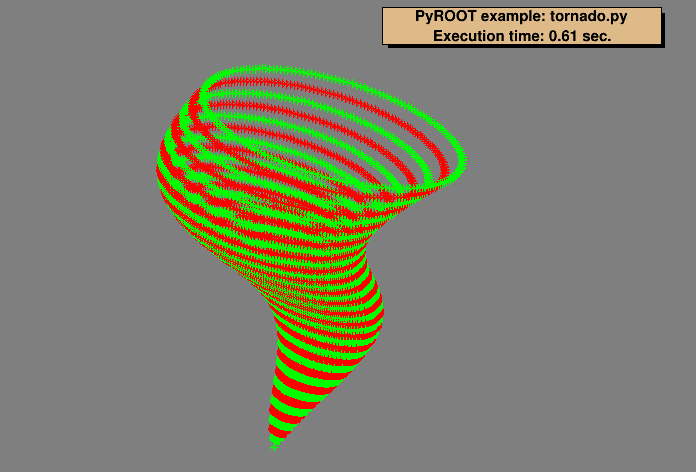Author: Wim Lavrijsen
This notebook tutorial was automatically generated with ROOTBOOK-izer from the macro found in the ROOT repository on Saturday, October 01, 2022 at 09:37 AM.

In :
from ROOT import TCanvas, TView, TPolyMarker3D, TPaveText
from ROOT import gROOT, gBenchmark
from math import cos, sin, pi

d = 16
numberOfPoints = 200
numberOfCircles = 40

Welcome to JupyROOT 6.27/01


create and open a canvas

In :
sky = TCanvas( 'sky', 'Tornado', 300, 10, 700, 500 )
sky.SetFillColor( 14 )


creating view

In :
view = TView.CreateView()
rng = numberOfCircles * d
view.SetRange( 0, 0, 0, 4.0*rng, 2.0*rng, rng )

polymarkers = []
for j in range( d, numberOfCircles * d, d ):

# create a PolyMarker3D
pm3d = TPolyMarker3D( numberOfPoints )

# set points
for i in range( 1, numberOfPoints ) :
csin = sin( 2*pi / numberOfPoints * i ) + 1
ccos = cos( 2*pi / numberOfPoints  * i ) + 1
esin = sin( 2*pi / (numberOfCircles*d) * j ) + 1
x = j * ( csin + esin );
y = j * ccos;
z = j;
pm3d.SetPoint( i, x, y, z );

# set marker size, color & style
pm3d.SetMarkerSize( 1 )
pm3d.SetMarkerColor( 2 + ( d == ( j & d ) ) )
pm3d.SetMarkerStyle( 3 )

# draw
pm3d.Draw()

# save a reference
polymarkers.append( pm3d )

ct = gBenchmark.GetCpuTime( 'tornado' )
timeStr = 'Execution time: %g sec.' % ct

text = TPaveText( 0.1, 0.81, 0.9, 0.97 )
text.SetFillColor( 42 )
text.Draw()

sky.Update()

tornado   : Real Time =   0.77 seconds Cpu Time =   0.61 seconds


Draw all canvases

In :
from ROOT import gROOT
gROOT.GetListOfCanvases().Draw()